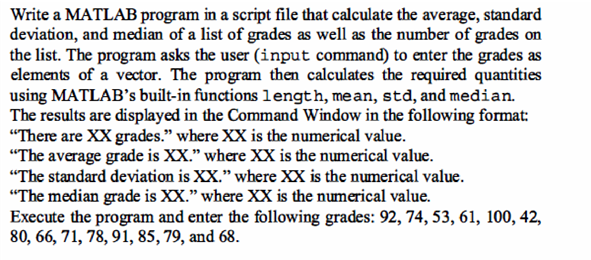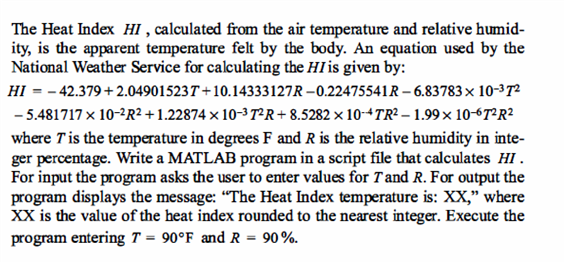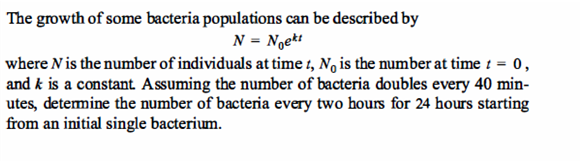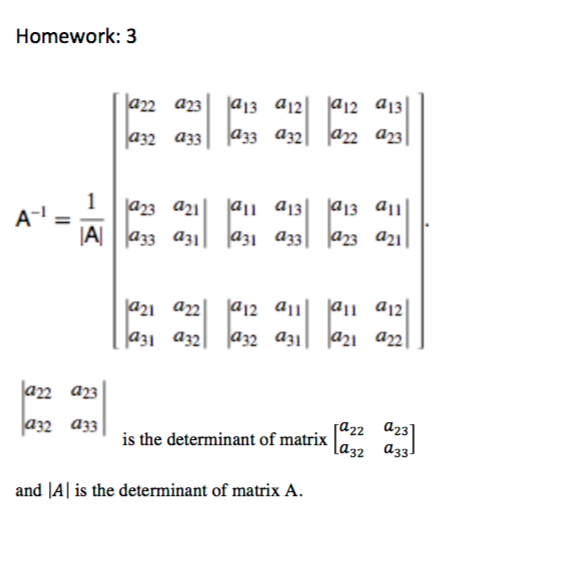# We Helped With This MATLAB Programming Homework: Have A Similar One?

SOLVEDCategory Programming MATLAB Undergraduate Solved Differential Equations Assignment Help Online

## Assignment Description

Dear Students,

Please find attached homework 4. Please write a separate .m file for each of the questions. For question

3 to 6 save the workspace as .mat file unless otherwise specified. Please add your name in the names of .mat and .m files (for eg. Question1_Suril.m Question1_suril.mat). This homework will be due at 11:55 PM on 10/27/2016.

Please let me know if you have any questions.

-Suril

Question 1. Write a MATLAB script to display following lines (use the function fprintf) (3 points).

1.       Suril had 25% in First quiz

2.       Suril worked ‘hard’ but had 25% in the first quiz

3.       AB is the same as B/A

Question 2. For the function fprintf explain the difference between notations %f, %e, %d and %s using an example. (2 points)

Question 3. Use the MATLAB program written in question 5 of homework 3 (matrix Inverse). Modify the program such that the matrix A is input by the user using ‘input’ command.

Question 4. Consider following set of linear equations (5 equations, 5 unknowns).  Where X1 to X5 are unknowns. A1 to E5 are coefficients and Y1 to Y5 are results. Write a MATLAB program to solve the equation. Ask user to input the values of A1 to E5 as matrix and Y1 to Y5 as secondary matrix.

Specifically ask user to input a 5 x 5 matrix and 1 x 5 matrix. (Use ‘input’) command.

A1*X1+B1*X2+C1*X3+D1*X4+E1*X5=Y1;

A2*X1+B2*X2+C2*X3+D2*X4+E2*X5=Y2;

A3*X1+B3*X2+C3*X3+D3*X4+E3*X5=Y3;

A4*X1+B4*X2+C4*X3+D4*X4+E4*X5=Y4; A5*X1+B5*X2+C5*X3+D5*X4+E5*X5=Y5;

Question 6. Write Matlab programs to solve following problems. (2 Points each) For each of the program also create a .mat file that will store the final output as described in each of the problems.

1.For question 1 save the workspace such that you are saving the values of input (grades) as well as mean, std, median. Information of length of variable is not necessary.

2.For question 2 Run program using 5 different values of T and R, which you can pick randomly. Store the output in the workspace file which will contain the heat index, T and R (as a separate column matrices) (1 point) and a combined matrix (3x5) where each column represents the values of T, R and heat index (1 point).

3.For question 3, save the whole workspace.

## Assignment ImageQ5. Pick a three dimension matrix A using randn(3,3). Calculate Inverse of the matrix (do not use inv(A) function in MATLAB). Show that Inverse calculated is correct using matrix multiplication. Matrix Inversion can be calculated using following method. (2 Points) For a matrix all a12 a13 A=a21 922 923 a31 a32 a33 The matrix inverse is

## Assignment ImageHomework: 3 A- = 1 A a22 923 a32 a33 a22 a23 a13 a12 a12 a13 a32 a33 a33 a32a22 923 a23a21 a11 a13 a13 a11 an a33 a31 a31 a33a23 921 921 922 912 911 911 912 also con a31 a32a32 931 a32 a31 a21 922 is the determinant of matrix and A is the determinant of matrix A. [a22 a23] [a32 a33]

Is it free to get my assignment evaluated?

Yes. No hidden fees. You pay for the solution only, and all the explanations about how to run it are included in the price. It takes up to 24 hours to get a quote from an expert. In some cases, we can help you faster if an expert is available, but you should always order in advance to avoid the risks. You can place a new order here.

How much does it cost?

The cost depends on many factors: how far away the deadline is, how hard/big the task is, if it is code only or a report, etc. We try to give rough estimates here, but it is just for orientation (in USD):

 Regular homework \$20 - \$150 Advanced homework \$100 - \$300 Group project or a report \$200 - \$500 Mid-term or final project \$200 - \$800 Live exam help \$100 - \$300 Full thesis \$1000 - \$3000

How do I pay?

Credit card or PayPal. You don't need to create/have a Payal account in order to pay by a credit card. Paypal offers you "buyer's protection" in case of any issues.

Why do I need to pay in advance?

We have no way to request money after we send you the solution. PayPal works as a middleman, which protects you in case of any disputes, so you should feel safe paying using PayPal.

Do you do essays?

No, unless it is a data analysis essay or report. This is because essays are very personal and it is easy to see when they are written by another person. This is not the case with math and programming.

Why there are no discounts?

It is because we don't want to lie - in such services no discount can be set in advance because we set the price knowing that there is a discount. For example, if we wanted to ask for \$100, we could tell that the price is \$200 and because you are special, we can do a 50% discount. It is the way all scam websites operate. We set honest prices instead, so there is no need for fake discounts.

Do you do live tutoring?

No, it is simply not how we operate. How often do you meet a great programmer who is also a great speaker? Rarely. It is why we encourage our experts to write down explanations instead of having a live call. It is often enough to get you started - analyzing and running the solutions is a big part of learning.

What happens if I am not satisfied with the solution?

Another expert will review the task, and if your claim is reasonable - we refund the payment and often block the freelancer from our platform. Because we are so harsh with our experts - the ones working with us are very trustworthy to deliver high-quality assignment solutions on time.

Customer Feedback

"Thanks for explanations after the assignment was already completed... Emily is such a nice tutor! "

Order #13073